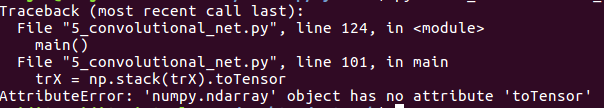# Converting the List of numpy image into torch tensor

Hi,

I was creating the data for CNN model using the following format:

``````## Get the location of the image and list of class

img_data_dir = "/Flowers"

## Get the contents in the image folder. This gives the folder list of each image "class"

contents = os.listdir(img_data_dir)

## This gives the classes of each folder. We will use these classes to classify each image type

classes = [each for each in contents if os.path.isdir(img_data_dir + "/" + each)]

batch = [] ## Empty list of image list
labels = []  ## Empty image labels list

for each in classes:  ## looping over each class

class_path = img_data_dir + "/" + each  ## create the path of each image class

files = os.listdir(class_path)  ## list of all files in each image class folder

for ii, file in enumerate(files, 1):  ## Enumerate over each image list

img = skimage.io.imread(os.path.join(class_path, file))  ## each the images from the folder. We are passing the file path + name in "imread"
img = img / 255.0 ## standardize the data

batch.append(img.reshape(1, 224, 224, 3))  ## reshaping images and append in one file list
labels.append(each) ## appending the labels of each image

trX, teX, trY, teY = train_test_split(batch, labels, test_size=0.2, random_state=0)
``````

But when I’m running the CNN model, I’m getting the following error:

Following this method, I tried

`trX = torch.stack(trX).float()`

But I was getting the following error:

Could anyone please help me how to convert list of images into tensor, so that I can incorporate it into CNN models?

Thank you!

PyTorch can only stack Torch tensors, use np.stack and then toTensor.

@mratsim: Thanks for your reply. I’m already stacking the images into numpy arrays. My problem is, how to convert this list into tensor values so that I can use in CNN.

I tried stack option as the link was suggesting. But this was just an experiment to solve my issue.

I don’t see the whole code unfortunately but I meant

trX = np.stack(trX).toTensor

trX = torch.stack(trX).float

According to your error message, when Torch tries to unsqueeze he gets a numpy.ndarray not a torch tensor

1 Like

But with your suggestion, I’m getting this error:What mratsim meant was to use `numpy.stack` to convert the list of 3D numpy array into a 4D Numpy array and then convert it a Torch Tensor using constructor, `from_numpy`, etc.

``````# Example
# I am assuming trX is a list of image arrays (1, 224, 224, 3)
# of length L = 0.8 * len(files)
>>> import numpy as np
>>> a = np.asarray(trX)
>>> a.shape # should be (L, 1, 224, 224, 3)
>>> a = np.squeeze(a, axis=1) # shape should now be (L, 224, 224, 3)
>>> import torch
>>> b = torch.floatTensor(a) # or torch.from_numpy(a)``````
2 Likes

Yes my bad, it’s been a while since I used PyTorch, trX = torch.from_numpy(np.stack(trX))

1 Like

Thank you to both Prasanna and Mamy! Your suggestion solves my issue. Actually I’m completely new to pytorch. So having issues with these small things.

Thanks again!